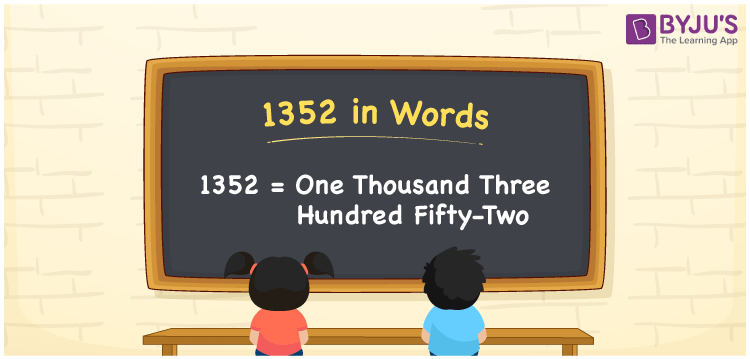# 1352 in Words

1352 in words can be written as One Thousand Three Hundred Fifty-Two. If the rent of a shed per month is Rs. 1352, then you can say that “The rent of a shed per month is One Thousand Three Hundred Fifty-Two Rupees”. Learn the place value concept anywhere and at any time using the materials given at BYJU’S. So, 1352 can be read as “One Thousand Three Hundred Fifty-Two” in words.

 1352 in words One Thousand Three Hundred Fifty-Two One Thousand Three Hundred Fifty-Two in Numbers 1352

## 1352 in English Words## How to Write 1352 in Words?

Learn the concept of writing numbers in words using the place value chart of 1352. The expanded form of 1352 helps students know the ones, tens, hundreds and thousand places.

 Thousands Hundreds Tens Ones 1 3 5 2

1352 in expanded form can be written as:

1 x Thousand + 3 × Hundred + 5 × Ten + 2 × One

= 1 x 1000 + 3 x 100 + 5 x 10 + 2 x 1

= 1000 + 300 + 50 + 2

= 1352

= One Thousand Three Hundred Fifty-Two

Hence, 1352 in words is written as One Thousand Three Hundred Fifty-Two.

1352 is a natural number that precedes 1353 and succeeds 1351.

1352 in words – One Thousand Three Hundred Fifty-Two

Is 1352 an odd number? – No

Is 1352 an even number? – Yes

Is 1352 a perfect square number? – No

Is 1352 a perfect cube number? – No

Is 1352 a prime number? – No

Is 1352 a composite number? – Yes

## Frequently Asked Questions on 1352 in Words

Q1

### How to write 1352 in words?

1352 can be written in words as “One Thousand Three Hundred Fifty-Two”.
Q2

### Write One Thousand Three Hundred Fifty-Two in numbers.

One Thousand Three Hundred Fifty-Two in numbers can be written as 1352.
Q3

### Is 1352 an even number?

1352 is an even number as it is completely divisible by 2.